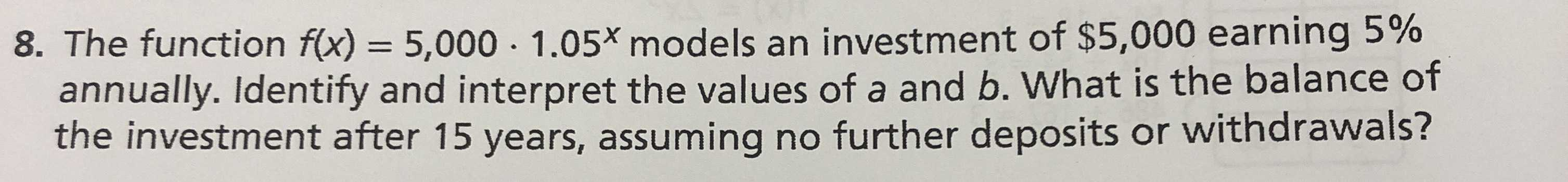### ¿Todavía tienes preguntas de matemáticas?

Pregunte a nuestros tutores expertos
Algebra
PreguntaThe function $$f ( x ) = 5,000 \cdot 1.05 ^ { x }$$ models an investment of $$\ 5,000$$ earning $$5 \%$$ annually. Identify and interpret the values of a and $$b .$$ What is the balance of the investment after $$15$$ years, assuming no further deposits or withdrawals?